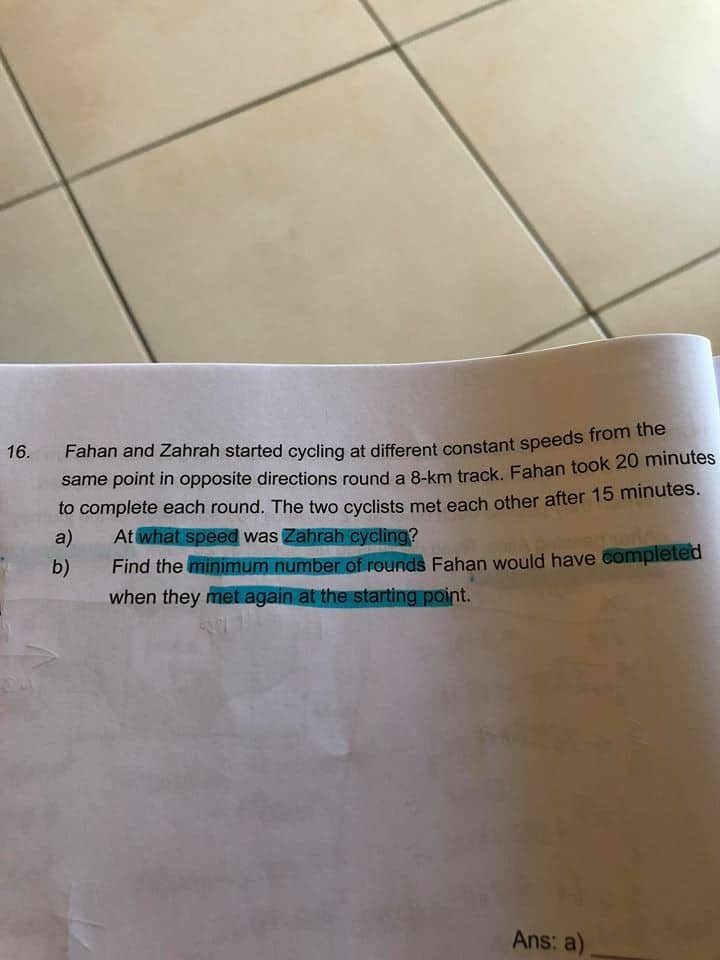# Question(a) F’s speed → 8km ÷ 1/3 hr

= 24 km/h

F’s distance travelled in 60 min → 24 km

F’s distance travelled in 15 min → 24 ÷ 4 = 8 km

Z’s distance travelled in 15 min → 8 – 6 = 2 km

Z’s speed → 2 ÷ 1/4 hr = 8km/h

(b) F takes 20 min to reach the starting point again.

Z takes 1hr (8km ÷ 8km/h) to reach the starting point again.

Therefore, F takes 1hr to complete 3 rounds and reach the starting point and meet Z. Thus the         minimum rounds for F is 3 rounds

Z’s distance travelled in 15 min → 8 – 6 = 2 km

how u got 6?

0 Replies 0 Likes

a) In 15 min, Farhan cycled 15/20*8 = 6 km.

Zahrah cycled 8 – 6 = 2 km.

Zahrah’s speed = 2 km ÷ 15/60 h = 8 km/h

b) Farhan completes each round in 20 min.

Zahrah completes each round in 60 min.

LCM of 60 and 20 = 60

They meet 1 hour after starting.

Farhan would have cycled 3 rounds.

0 Replies 0 Likes